# How to convert metric to imperial - and back again

Last update: 02 November 2013

Metric measures are always easy to work with - particularly if you have a calculator. But what if you need to convert Metric units like kilometres, kilograms or litres to or from Imperial units like miles, pounds or pints? And what if you need to do it right now, in your head?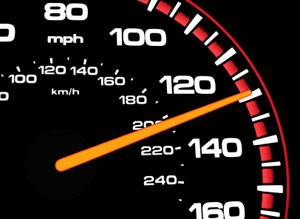The good news is that it is possible - even easy - to convert Metric weights and measures to Imperial ones provided you're prepared to think creatively about how to approach the problem. Looking at "mathsmadeeasy.com" type websites won't necessarily help you with this since they tend to say things like: "Take your measure. Multiply by the conversion factor. Write down the answer." Brilliant.

With all these Imperial/Metric conversions, there's usually a trade off between speed and accuracy. If you really must have an answer that's correct to two or three places of decimals, you will need the actual conversion factors and a calculator. If you're prepared to accept a 'close enough' guide figure, then you can do these in your head. Sometimes, though, the answers can be remarkably precise.

Let's demonstrate this with conversions for miles and kilometers, pounds and kilos, liters and imperial gallons and liters and US gallons

## Miles and kilometers

The standard 'converter' approach applied to the common problem of turning, say, 144 kilometres into miles would require you to remember that one km equals 0.62137119 miles and being able to multiply that by 144 in your head. Well, good luck with that. Rain Man might be able to do it. I certainly can't.

What I can do is to remember that, more sensibly, five miles equals eight kilometres and to use a trick an uncle taught me on an early drive through France.

You take the kilometre number, 144, and halve it; 72. Then take a quarter of your half, 18, and just add the two together. 72 + 18 = 90. That's your answer in miles.

What about going the other way, for the European traveller trying to convert those weird miles into nice neat kilometres? Well, the obvious way is to divide by five and then multiply by eight. 90 ÷ 5 = 18 x 8 = 144.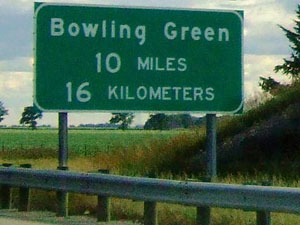So, yes, of course it works but there's a problem; While dividing by five is easy enough (divide by ten and double it), multiplying by eight is trickier. One way to solve it is to reduce the principal number to something that appears in the eight times table then convert it back. So that 18 could be halved to nine and we all remember, don't we, that nine eights are 72. Double back again and we get to 144.

Alternatively, let's think about about a kilometre equalling (roughly) six tenths of a mile. This is why British and American soldiers stationed in Germany were taught an 'on manoeuvres' converter: divide the miles by six and move the decimal point one point to the right.

Let's try it. Six goes into 90 fifteen times. Express that as 15.0 and move the decimal point one place right and arrive at 150. You can also divide by three and multiply by five: 90 ÷ 3 = 30 x 5 = 150. Pretty close (but I hope they don't use it for calculating artillery ranges).

Again, though, there's a formula that's almost as easy to work out in your head but delivers a precise result. Divide the number of miles by five and subtract that from the original. Then double the resulting number. So:

90 miles ÷ 5 = 18.
90 - 18 = 72.
72 x 2 = 144 kilometers.

That'll do nicely. Did you see what we did there? Turned the miles number into four fifths and then doubled it to make eight fifths, or the reverse of five eighths. Sometimes fractions are easier than decimals.

## Pounds and kilos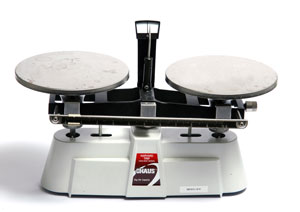Well, the officlal conversion factor may be 1lb equals 0.45359237 kg, but it's much easier to remember one kilo equals about 2.2 pounds either side of the Atlantic. So, take your kilos, double them, divide the answer by ten, add the two numbers together and there's your answer in pounds.

Let's try converting 4.5 kilos: We double that to nine kilos than add a tenth of nine, which is 0.9. and arrive at 9.9 lbs. How accurate is that? Well, multiplying 4.5 by the official conversion factor for US pounds would give us 9.9208018 lbs, so this converter works very well.

And going the other way, you halve your pounds, then subtract one tenth of the result for a kilos total.

So 9.9 lbs halved is 4.95. Subtract 0.49 from that and you arrive at 4.46, which is close enough.

Should you wish to use an online tool for this conversion, you can use our mass and weight converter.

## Liters and Imperial gallons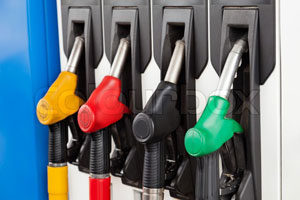Converting litres to gallons and back again is a conversion we're all having to do more often in these days when motor manufacturers express fuel economy figures in miles per gallon to make the figures sound more impressive, while fuel is now priced in litres to make the prices seem slightly less scary.

The basic conversion factors here are that one litre equals 1.8 Imperial pints and there are eight pints in a gallon. Therefore one gallon equals 4.55 litres, while one litre equals 0.264 gallons. Fine, if you have a calculator...

If not, then the method is to multiply your gallons by nine then halve it. Let's try that with eight Imperial gallons.

8 x 9 = 72
72 ÷ 2 = 36.

The precise conversion is that eight Imperial gallons equals 36.36872 litres, so this is accurate enough for most purposes.

Going the other way, double the litres, then divide by nine and finally round down to just one place of decimals.Thus 25 litres would be:

25 x 2 = 50
50 ÷ 9 = 5.55, translating to five and a half gallons. The actual figure is 5.499 gallons so, again, this is a useful method.

## Liters and US gallons

Why is the US gallon smaller than the Imperial one, equivalent to 3.78 liters? Personally, I blame the American Revolution. The USA is still using the original gallon from the days of Queen Anne. Following the rather messy divorce from the Crown in 1775-1783, the Yankees seemed a little sniffy about having anything to do with the British reform of Imperial Weights and Measures that happened in 1824.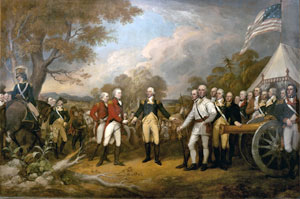Well, that's history, but it means we need a different conversion to turn liters into US gallons (USG), and vice versa. One litre equals 2.1 US pints, so one way to do it would be double the liters figure, add one twentieth to the figure and divide by eight to find the USG total.

Thus 25 liters doubled would be 50. Add 2.5, to make 52.5 and divide by eight to reach 6.6 USG. (Official conversion: 6.6043013).

To go the other way, multiply the USGs by eight; take one-twentieth off the total and then halve the result. So, eight gallons would translate as 8 x 8 = 64, minus 3.2, equals 60.8, halved, to give us 30.4 liters, compared with exact conversion of 30.28 liters.

You might be tempted to reduce the USG total by one-fifth to give you Imperial gallons and convert into liters that way. My advice here would be "Citizen, Beware." Following this method will give you a result of USG 8 = 28.8 liters, which is way off.

This just shows the danger of combining two or more 'rough and ready' formulas into the same conversion. Sometimes, if you're lucky, the small variances cancel each other out. More often, as here, minor errors compound each other into one major one.

Of course, should you wish to use an online converter to check your answers then we have a liters to US gallons converter here.

Written by Nick Valentine